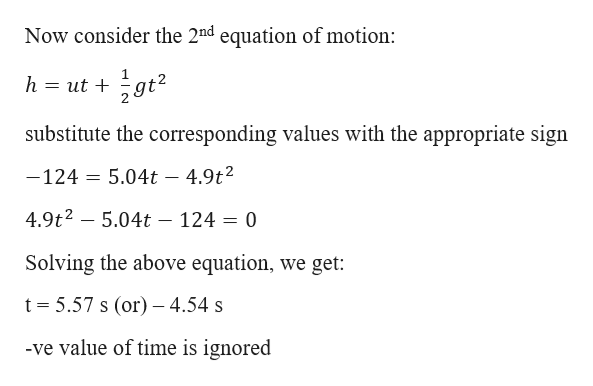# A helicopter is ascending vertically with a speed of 5.04 m/s . At a height of 124 m above the Earth, a package is dropped from the helicopter. How much time does it take for the package to reach the ground? [Hint: What is v0 for the package?] Express your answer to three significant figures and include the appropriate units.

Question

A helicopter is ascending vertically with a speed of 5.04 m/s . At a height of 124 m above the Earth, a package is dropped from the helicopter. How much time does it take for the package to reach the ground? [Hint: What is v0 for the package?] Express your answer to three significant figures and include the appropriate units.

check_circleExpert Solution
Step 1

Given information:

Vertical velocity of the helicopter (u) = 5.04 m/s

Height of the helicopter (h) = 124 m

Let “t” be the time taken for the package to reach the ground

We know that value of “g” is 9.81 m/ s2

Consider upward direction as “+ve” and down ward direction as “-ve”

Step 2

The initial velocity of the package will be equal ...help_outlineImage TranscriptioncloseNow consider the 2nd equation of motion: h = ut + substitute the corresponding values with the appropriate sign 5.04t 4.9t2 -124 4.9t2 -5.04t - 124 = 0 Solving the above equation, we get: t 5.57 s (or) 4.54 s -ve value of time is ignored fullscreen

### Want to see the full answer?

See Solution

#### Want to see this answer and more?

Solutions are written by subject experts who are available 24/7. Questions are typically answered within 1 hour*

See Solution
*Response times may vary by subject and question
Tagged in

### Kinematics